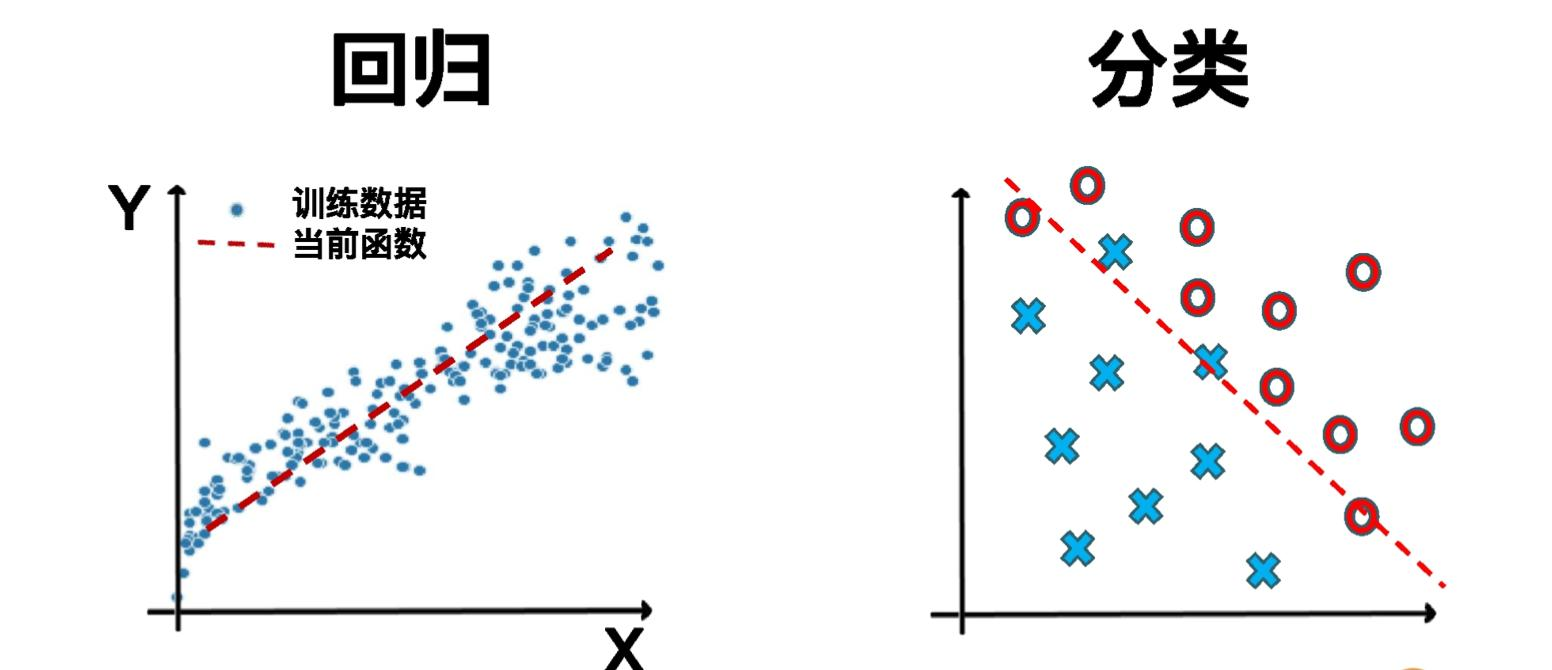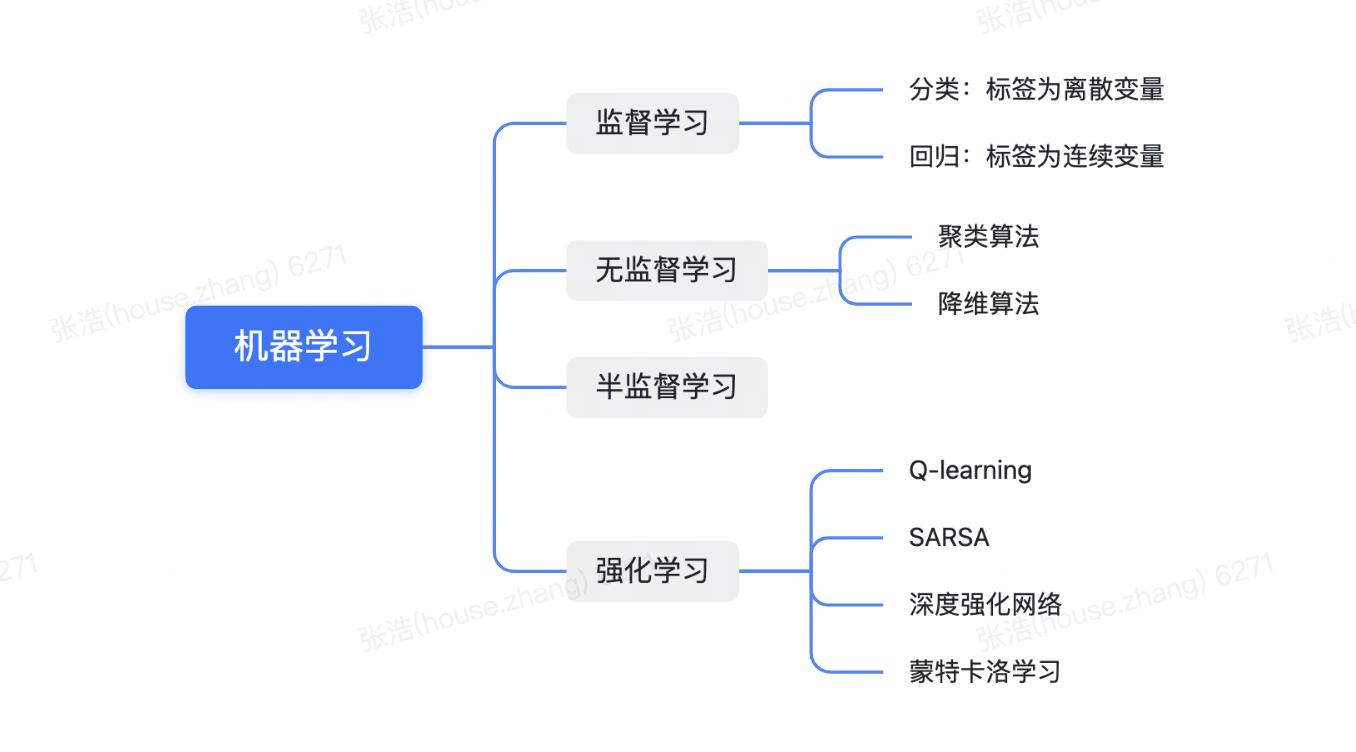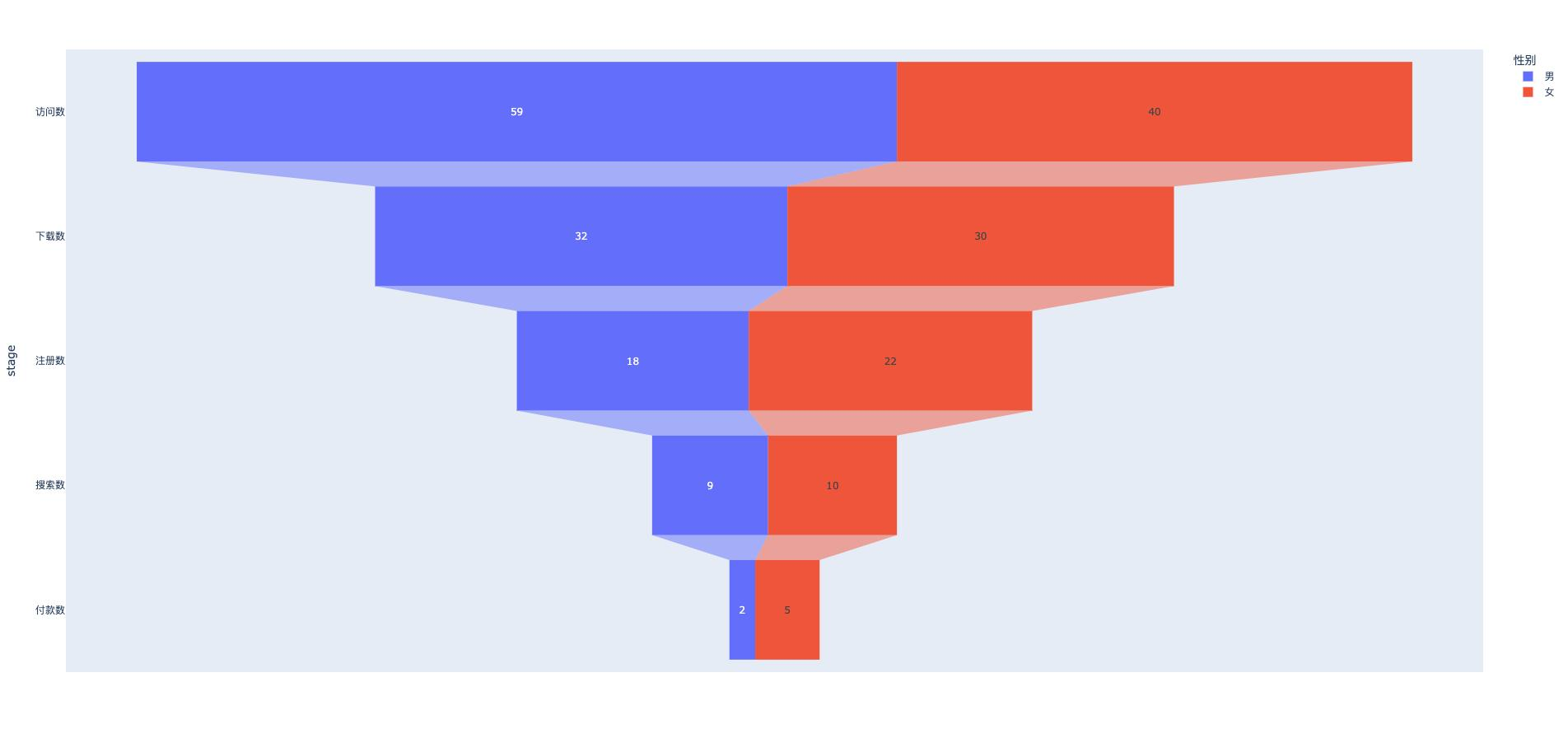# 浅谈AI机器学习及实践总结 | 社区征文## 机器学习分类

• 监督学习：训练的数据集全部都有标签，根据标签的特点 监督学习可以分为两类问题：回归和分类，回归问题的标签是连续的数值，比如预测房价、股市等，分类问题的标签是离散的数值，比如人脸识别、判断是否正确等，判断两款运营策略哪种更有效。• 无监督学习：训练数据集没有标签，多应用在聚类、降维等有限的场景中，比如说为用户做分组画像，另外通常也会作为数据预处理的一个子步骤中。

• 半监督学习：有的数据有标签、有的数据没有标签。往往是因为获取数据标签的难度很高，半监督学习与监督学习是很相似的，主要在与多了伪标签生成环节，也就是给无标签的数据人工 贴标签。

• 强化学习：针对于一些既不能用监督学习也不能用半监督和无监督学习来解决，这时候强化学习就上场了，它针对是智能体（可以理解成一种机器学习模型）如何基于环境而做出行动反应，以获得最大化的累积奖励。其与监督学习的差异在于监督学习是从数据中进行学习，而强化学习是从环境给他的奖惩中学习。

Q-learning,SARSA,深度强化网络、蒙特卡洛学习...## 背景：

jupyter notebook 可以交互式的开发，再加上拥有丰富的的文本格式、可以图文并茂的展示结果，迅速的展现数据分析师的想法。

## 安装Jupyter Notebook

### Anaconda 安装管理Juypter Notebook

Anaconda是一个免费的开发环境，能帮你管理众多的Python库，支持Jupyter Notebook、Spyder等工具，还有许多科学包，通过可以从官网上直接下载安装Anaconda，启动Anaconda后 安装Juypter就比较简单，直接Anaconda界面上启动就好了，默认Anaconda会安装好Juypter和相关科学库。

### 使用pip命令安装

``````pip3 install --upgrade pip ## 更新
pip3 install jupyter  ## 安装
jupyter notebook --port <port_number>  ## 启动可以指定端口号，不指定默认8888 当
# 还可以指定其他参数具体可以 jupyter notebook -h
``````

### 使用Docker安装

docker安装启动jupyter就比较简单了

## 实践Juypter notebook

### 在Juypter中使用Plotly 绘图

#### 简介

Plotly 是一个非常强大的开源数据可视化框架，它通过构建基于 HTML 的交互式图表来显示信息，可创建各种形式的精美图表。本文所说的 Plotly 指的是 Plotly.js 的 Python 封装，plotly本身是个生态非常复杂的绘图工具，它对很多编程语言提供接口，交互式和美观易用应该是 Plotly 最大的优势

### 绘制流程

• 安装Plotly包
``````pip install plotly
``````
• 详细代码

``````
import plotly.express as px # 导入需要的模块，命名为px
import pandas as pd

stages = ["访问数", "下载数", "注册数", "搜索数", "付款数"]
#漏斗的数据
data = pd.DataFrame(dict( #准备漏斗数据
number=[59, 32, 18, 9, 2],
stage=stages))
data['性别']='男'
print(data)

data2 = pd.DataFrame(dict( #准备漏斗数据
number=[40, 30, 22, 10, 5],
stage=stages))

data2['性别']='女'

df = pd.concat([data,data2],axis=0) # 拼接漏斗数据，pandas函数拼接支持DataFrame类型
print(df)
fig = px.funnel(df,x='number',y='stage',color='性别') #把数据传入漏斗
fig.show()  # 显示漏斗数据
``````
• 结果显示• 结果分析

## 收集数据和预处理

1. 收集数据

1. 数据可视化

``````#加载数据
import pandas as pd # 导入pandas数据处理工具包
``````

python数据可视化必备工具包：一个是python画图工具库Matplotlib，另外一个是统计学数据可视化工具Seaborn。

``````#散点图来观察数据之间分布关系
import matplotlib.pyplot as plt
import seaborn as sns
plt.xlabel('goods')
plt.ylabel('views')
plt.legend()
plt.show()
````````````
fig = sns.boxplot(x='热度指数', y="浏览量", data=data) # 用seaborn的箱线图画图
fig.axis(ymin=0, ymax=800000); #设定y轴坐标
``````
1. 数据清洗

• 第一种是处理缺失的数据，如果备份系统里面有缺了的数据，尽量去补录。如果没有可以剔除残缺的数据，也可以用其他数据记录的平均值、随机值或者0来补值，这个补值的过程叫数据修复。
• 第二种是处理重复的数据，如果完全重复的数据删掉就行，如果同一个主键出现两行不同的数据，就需要看看有没有其他辅助的信息可以帮助我们判断（如时间戳），要是无法判断的话，只能随机删除或者全部保留。
• 第三种是处理错误数据：比如商品的销售量、销售金额出现负值，这时候需要删除或者转化为有意义的值，再比如表示百分比或者概率的字段，如果值大于1，也属于逻辑错误数据
• 第四种处理不可用的数据，这指的是整理数据的格式，比如有些商品是以人民币为单位，有些以美元为单位，就需要线统一，另外就是把是和否转换为1、0 值再输入机器学习模型。

``````   df_ads.isna().sum() # Nan出现的个数。
``````

``````df_ads = df_ads.dropna()#把出现了NaN的数据行删掉
``````

1. 特征工程

1. 构建特征集和标签集

``````X=df_ads.drop['浏览量'],axis=1)：
``````

1. 拆分训练集、验证集和测试集合

``````from sklearn.model_selection import train_test_split
X_train,X_test,y_train,y_test = train_test_split(X,y,test_size=0.2,random_state=0)
``````## 选择算法和训练模型

Y = w*x +b

### 建立模型

``````from sklearn.liner_model import LinerRegression # 导入线性回归算法模型
linereg_model = LinearRegression() #    使用线性回归创建模型
``````

fit_intercept ，默认值为True，代表是否计算模型的截距

normalize，默认值为Flase代表是否对特征X在回归之前做规范化。

## 训练拟合模型

``````linereg_model.fit(x_train,y_train) # 用训练集数据，训练机器，拟合函数，确定内部参数

``````

## 评估并优化模型性能

``````y_pred = linereg_model.predict(x_test) #预测测试集的Y值
``````

``````df_ads_pred= X_test.copy() #测试集特征数据
``````

``````linereg_model.coef_
linereg_model.intercept_
``````

``````  linears_model.score(x_test,y_test)
``````

## 模型在线服务

• 预存推荐结果

• 利用 PMML 转换和部署模型

PMML（预测模型标记语言）：JPMML 作为序列化 xml 和解析 PMML 文件的 Library 库

• TensorFlow Serving

Tesorflow 模型上线流程主要是，先离线把模型序列化存储到文件系统，Tensorflow Serving 把模型文件载入到模型服务器，还原模型推断过程，对外以 HTTP 接口或 gRPC 接口的方式提供模型服务

https://zhuanlan.zhihu.com/p/74874291

https://zhuanlan.zhihu.com/p/33794257

637
1
0
0